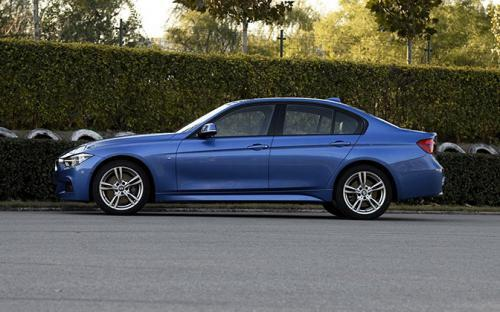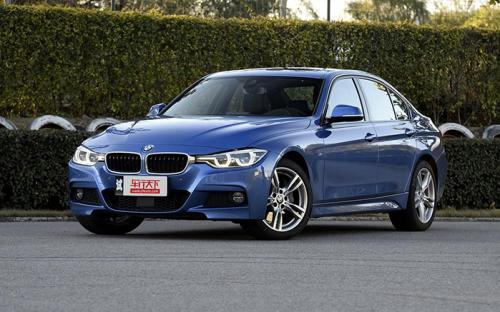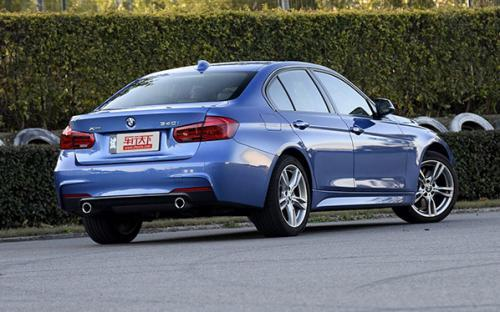### 宝马(进口) 宝马3系(进口)2017款最低售价：65.39 万元起

4650(mm)1811(mm)1454(mm)##### 配置亮点：
• 胎压监测装置

• ISOFIX儿童座椅接口

• 车身稳定控制(ESC/ESP/DSC等)

• 电动天窗

• 定速巡航

• 后倒车雷达

• 真皮座椅

• GPS导航系统

• 氙气大灯

• 后视镜加热

• 提交
2017款 340i xDrive M运动型 (116张)

宝马(进口) 宝马3系(进口) 绕车实拍• 宝马(进口) 宝马3系(进口) 在售车型

排量 车型 厂商指导价 本地最低报价 购车工具
T
340i xDrive M运动型 8挡手自一体
68.40万
65.39万
3.01万

宝马(进口) 宝马3系(进口) 经销商

查看更多 >>

### 宝马(进口) 宝马3系(进口) 动力加速

宝马3系(进口) 0-100公里加速时间分布在 4.9-4.9秒 属于 高性能级

动力级别 加速时间 车型
高性能级(1款)
4.9s
340i xDrive M运动型

宝马(进口) 宝马3系(进口) 视频

宝马(进口) 宝马3系(进口) 新闻资讯

# 纯电续航35km 3系插电式混动版参数公布

新闻 超过3127次关注

日前，宝马公布了3系plug-inhybrid插电式混合动力版的动力参数信息，该车综合最大输出功率为245马力（180kW），纯电模式下续航里程为35公里，综合油耗达到2升/100公里。

# 340i取代335i车型 宝马新款3系新消息

新闻 超过3747次关注

海外媒体近期报道称，宝马将于2015年正式推出新款3系车型，其中新款3系将针对目前细分车型进行调整，其中“335i”车型将由“340i”车型取代。据悉，未来新款3系（34...

# 配高功率发动机 疑似宝马340i谍照曝光

新闻 超过2625次关注

在12月初，海外媒体曾报道关于宝马计划推出一款340i车型来取代现款335i的消息。而在近日，疑似该车型的谍照在网络上曝光出来，另外海外媒体还有一种猜测是，谍照中...

# 宝马召回15474辆老款3系 安全气囊缺陷

碰撞召回 超过6697次关注

日前，宝马（中国）汽车贸易有限公司、华晨宝马汽车有限公司根据《缺陷汽车产品召回管理条例》的要求，向国家质检总局备案了召回计划，决定自2014年8月25日起，召回...

# BMW mission3行动再传帖 问天下谁是英雄

行业动态 超过5221次关注

无论是运动场的英雄还是职场精英，现在有一项比赛可以让你发现一个完全不同的自我：2014BMW3行动郑州站于8月1日—8日举行。只要能通过心智、体能和驾驶技术三项挑战...

# 中国同步 亚琛施耐泽推出高效动力计划

车系文章 超过2647次关注

琛施耐泽（ACSchnitzer）作为宝马改装专家，其产品几乎覆盖了宝马整个车系。近日，亚琛施耐泽推出了高效动力计划，针对搭载N13、N20发动机高功率版的宝马1系和3系车...

# 华丽丽的换代 全新宝马3系官图解析

试驾评测 超过4109次关注

将宝马3系称为运动轿车的标杆一点都不为过，这不仅仅是一个口号，也是积累的口碑，完美的前后质量配比、精准的转向、出色底盘调教功底，宝马3系从设计初衷到实际表...

# 外观造型更运动 实拍宝马328i M运动型

专业导购 超过4344次关注

如果你嫌宝马328i运动设计套装车型的外观运动得还不够彻底，正思绪着怎么改装。在去年广州车展上，宝马新推出的328iM运动型或许能让您满意，它在328i运动设计套装车...

# 售32-66.9万 2014款3系标准轴距版上市

车系文章 超过2223次关注

2014款3系标准轴距版的大部分车型标配了升级的iDriver系统。另外，与2013款不同的是320i运动设计套装及以上车型（ActiveHybrid3除外）均取消了国产版本，只有进口版车型可以选择。

# 宝马3系(进口）旅行版赠牌照与首年保险

车系文章 超过2289次关注

近日，编辑从宝马品牌授权经销商天津中顺津宝5S店了解到，宝马3系（进口）少量现车有售，购旅行版车型可赠送牌照和首年保险，感兴趣的网友可到店内试驾，详情可咨询...

# 含3系/5系等 宝马将在华召回超20万辆车

车系文章 超过2217次关注

日前，宝马（中国）汽车贸易有限公司、华晨宝马汽车有限公司根据《缺陷汽车产品召回管理条例》的要求，向国家质检总局备案了召回计划，决定自2014年6月18日起，召回...

猜你喜欢

﻿
• 快速找车
• 选择品牌
• 选择品牌
• A  奥迪
• A  阿斯顿·马丁
• A  阿尔法·罗密欧
• B  宝沃
• B  布加迪
• B  巴博斯
• B  保时捷
• B  宾利
• B  奔驰
• B  宝马
• B  本田
• B  别克
• B  标致
• B  比亚迪
• B  宝骏
• B  北汽制造
• B  北汽新能源
• B  北汽幻速
• B  北汽威旺
• B  北京汽车
• B  奔腾
• B  北汽绅宝
• C  长安
• C  长安商用
• C  长城
• C  昌河
• D  大众
• D  道奇
• D  DS
• D  东南
• D  东风风神
• D  东风风行
• D  东风小康
• D  东风风度
• D  东风
• F  福特
• F  丰田
• F  菲亚特
• F  法拉利
• F  福田
• F  福迪
• F  福汽启腾
• G  观致
• G  广汽传祺
• G  广汽吉奥
• G  GMC
• H  红旗
• H  汉腾汽车
• H  哈弗
• H  哈飞
• H  海格
• H  海马
• H  华颂
• H  黄海
• H  华泰
• H  恒天
• J  吉利汽车
• J  捷豹
• J  Jeep
• J  江淮
• J  江铃
• J  金杯
• J  九龙
• J  金旅
• K  凯翼
• K  凯迪拉克
• K  克莱斯勒
• K  科尼塞克
• K  卡威
• K  开瑞
• L  路虎
• L  林肯
• L  劳斯莱斯
• L  兰博基尼
• L  雷克萨斯
• L  铃木
• L  雷诺
• L  理念
• L  力帆
• L  莲花汽车
• L  猎豹
• L  路特斯
• L  陆风
• M  马自达
• M  MG
• M  MINI
• M  玛莎拉蒂
• M  摩根
• M  迈凯轮
• N  纳智捷
• O  欧宝
• O  讴歌
• O  欧朗
• Q  奇瑞
• Q  起亚
• Q  启辰
• R  日产
• R  荣威
• R  瑞麒
• S  三菱
• S  斯威汽车
• S  萨博
• S  smart
• S  斯柯达
• S  斯巴鲁
• S  思铭
• S  双龙
• S  上汽大通
• S  双环
• T  特斯拉
• T  腾势
• W  沃尔沃
• W  五菱汽车
• W  五十铃
• W  威兹曼
• W  威麟
• X  现代
• X  雪佛兰
• X  雪铁龙
• X  西雅特
• Y  一汽
• Y  英菲尼迪
• Y  英致
• Y  依维柯
• Y  野马汽车
• Y  永源
• Z  众泰
• Z  中华
• Z  中兴
• Z  知豆
• 选择车系
• 选择车系
• 车型对比
• 选择品牌
• 选择品牌
• A  奥迪
• A  阿斯顿·马丁
• A  阿尔法·罗密欧
• B  宝沃
• B  布加迪
• B  巴博斯
• B  保时捷
• B  宾利
• B  奔驰
• B  宝马
• B  本田
• B  别克
• B  标致
• B  比亚迪
• B  宝骏
• B  北汽制造
• B  北汽新能源
• B  北汽幻速
• B  北汽威旺
• B  北京汽车
• B  奔腾
• B  北汽绅宝
• C  长安
• C  长安商用
• C  长城
• C  昌河
• D  大众
• D  道奇
• D  DS
• D  东南
• D  东风风神
• D  东风风行
• D  东风小康
• D  东风风度
• D  东风
• F  福特
• F  丰田
• F  菲亚特
• F  法拉利
• F  福田
• F  福迪
• F  福汽启腾
• G  观致
• G  广汽传祺
• G  广汽吉奥
• G  GMC
• H  红旗
• H  汉腾汽车
• H  哈弗
• H  哈飞
• H  海格
• H  海马
• H  华颂
• H  黄海
• H  华泰
• H  恒天
• J  吉利汽车
• J  捷豹
• J  Jeep
• J  江淮
• J  江铃
• J  金杯
• J  九龙
• J  金旅
• K  凯翼
• K  凯迪拉克
• K  克莱斯勒
• K  科尼塞克
• K  卡威
• K  开瑞
• L  路虎
• L  林肯
• L  劳斯莱斯
• L  兰博基尼
• L  雷克萨斯
• L  铃木
• L  雷诺
• L  理念
• L  力帆
• L  莲花汽车
• L  猎豹
• L  路特斯
• L  陆风
• M  马自达
• M  MG
• M  MINI
• M  玛莎拉蒂
• M  摩根
• M  迈凯轮
• N  纳智捷
• O  欧宝
• O  讴歌
• O  欧朗
• Q  奇瑞
• Q  起亚
• Q  启辰
• R  日产
• R  荣威
• R  瑞麒
• S  三菱
• S  斯威汽车
• S  萨博
• S  smart
• S  斯柯达
• S  斯巴鲁
• S  思铭
• S  双龙
• S  上汽大通
• S  双环
• T  特斯拉
• T  腾势
• W  沃尔沃
• W  五菱汽车
• W  五十铃
• W  威兹曼
• W  威麟
• X  现代
• X  雪佛兰
• X  雪铁龙
• X  西雅特
• Y  一汽
• Y  英菲尼迪
• Y  英致
• Y  依维柯
• Y  野马汽车
• Y  永源
• Z  众泰
• Z  中华
• Z  中兴
• Z  知豆
• 选择车系
• 选择车系
• 选择车型
• 选择车型
• 意见反馈